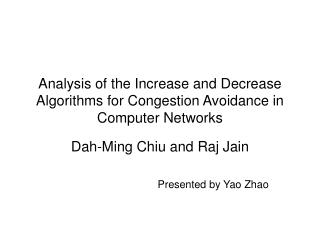Download PresentationAnalysis of the Increase and Decrease Algorithms for Congestion Avoidance in Computer Networks

# Analysis of the Increase and Decrease Algorithms for Congestion Avoidance in Computer Networks - PowerPoint PPT PresentationDownload Presentation## Analysis of the Increase and Decrease Algorithms for Congestion Avoidance in Computer Networks

- - - - - - - - - - - - - - - - - - - - - - - - - - - E N D - - - - - - - - - - - - - - - - - - - - - - - - - - -
##### Presentation Transcript

1. Analysis of the Increase and Decrease Algorithms for Congestion Avoidance in Computer Networks Dah-Ming Chiu and Raj Jain Presented by Yao Zhao

2. Motivation (1) • Internet is heterogeneous • Different bandwidth of links • Different load from users • Congestion control • Help improve performance after congestion has occurred • Congestion avoidance • Keep the network operating off the congestion

3. Motivation (2) • Fig. 1. Network performance as a function of the load. Broken curves indicate performance with deterministic service and interarrival times

4. Relate Works • Centralized algorithm • Information flows to the resource managers and the decision of how to allocate the resource is made at the resource [Sanders86] • Decentralized algorithms • Decisions are made by users while the resources feed information regarding current resource usage [Jaffe81, Gafni82, Mosely84] • Binary feedback signal and linear control • Synchronized model • What are all the possible solutions that converge to efficient and fair states

5. Control System

6. Linear Control (1) • 4 examples of linear control functions • Multiplicative Increase/Multiplicative Decrease • Additive Increase/Additive Decrease • Additive Increase/Multiplicative Decrease • Additive Increase/ Additive Decrease

8. Criteria for Selecting Controls • Efficiency • Closeness of the total load on the resource to the knee point • Fairness • Users have the equal share of bandwidth • Distributedness • Knowledge of the state of the system • Convergence • The speed with which the system approaches the goal state from any starting state

9. Responsiveness and Smoothness of Binary Feedback System • Equlibrium with oscillates around the optimal state

10. Vector Representation of the Dynamics

11. Convergence to Efficiency • Negative feedback • So • Or

12. c>0 Convergence to Fairness (1) where c=a/b (6)

13. Convergence to Fairness (2) • c>0 implies: • Furthermore, combined with (3) we have:

14. Distributedness • Having no knowledge other than the feedback y(t) • Each user tries to satisfy the negative feedback condition by itself • Implies (10) to be

15. Truncated Case

16. Important Results • Proposition 1: In order to satisfy the requirements of distributed convergence to efficiency and fairness without truncation, the linear increase policy should always have an additive component, and optionally it may have a multiplicative component with the coefficient no less than one. • Proposition 2: For the linear controls with truncation, the increase and decrease policies can each have both additive and multiplicative components, satisfying the constrains in Equations (16)

17. Vectorial Representation of Feasible conditions

18. Optimizing the Control Schemes • Optimal convergence to Efficiency • Tradeoff of time to convergent to efficiency te, with the oscillation size, se. • Optimal convergence to Fairness

19. Optimal convergence to Efficiency • Given initial state X(0), the time to reach Xgoal is:

20. Optimal convergence to Fairness • Equation (7) shows faireness function is monotonically increasing function of c=a/b. • So larger values of a and smaller values b give quicker convergence to fairness. • In strict linear control, aD=0 => fairness remains the same at every decrease step • For increase, smaller bI results in quicker convergence to fairness => bI =1 to get the quickest convergence to fairness • Proposition 3: For both feasibility and optimal convergence to fairness, the increase policy should be additive and the decrease policy should be multiplicative.

21. Practical Considerations • Non-linear controls • Delay feedback • Utility of increased bits of feedback • Guess the current number of users n • Impact of asynchronous operation

22. Conclusion • We examined the user increase/decrease policies under the constrain of binary signal feedback • We formulated a set of conditions that any increase/decrease policy should satisfy to ensure convergence to efficiency and fair state in a distributed manner • We show the decrease must be multiplicative to ensure that at every step the fairness either increases or stays the same • We explain the conditions using a vector representation • We show that additive increase with multiplicative decrease is the optimal policy for convergence to fairness